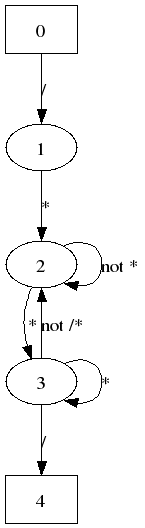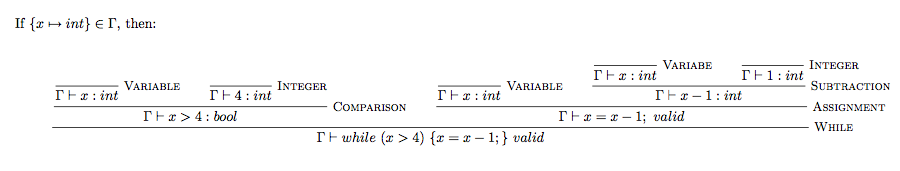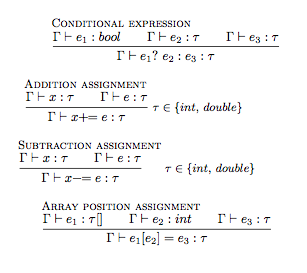# Solutions to Selected Exercises

Implementing Programming Languages (Programming Language Technology Course 2007-2012)
Aarne Ranta, Björn Bringert, et al.

1.

```    int main ()
{
int i ;
int j = k + 1 ;          // unknown variable k: type checker
int a[] = {1,2,3}        // missing semicolon: parser
j = a + 6 ;              // addition of integer to array: type checker
a = 7 ;               // too big array index: runtime
printf("hello world\n) ; // unterminated string: lexer
// no return of type int: type checker
}
```

2.

```    0001 0000 1111 1111  bipush   -1
0001 0000 0010 0000  bipush   32
0110 0100            isub
0001 0000 0000 1001  bipush    9
0110 1000            imul

(-1 - 32) * 9
```

3.

```  while (x > y) y = x = 3 ;

AST:
SWhile (EGt (EIdent (Ident "x")) (EIdent (Ident "y")))
(SExp (EAss (Ident "y") (SExp (EAss (Ident "x") (EInt 3)))))

```

4. Numerals

```    N0, NP.       Num ::= "0" | Pos
PD, PPD, PP0. Pos ::= Dig | Pos Dig | Pos "0"
D1,...        Dig ::= "1" | "2" | "3" | "4" | "5" | "6" |"7" | "8" | "9"

NP (PPD (PP0 (PP0 (PD D2))) D7)
```

5. Unix

```  Script  ::= [Line] ;
Line    ::= [Command] In Out ;
In      ::= "<" Ident | ;
Out     ::= ">" Ident | ;
Command ::= Ident [Opt] [Arg] ;
Arg     ::= File ;
Opt     ::= "-" Ident ;

[Opt]   ::= Opt [Opt] | ;
[Arg]   ::= Arg [Arg] | ;

[Command] ::= Command "|" [Command] | Command ;
```

6. Lisp

```  entrypoints Program;

Prog.   Program ::= [Def] ;
terminator Def "";

Defun.  Def ::= "(" "defun" LIdent "(" [LIdent] ")" Expr ")" ;
separator LIdent "" ;

EApp.   Expr ::= "(" [Expr] ")" ;
EAtom.  Expr ::= LIdent ;
EQuote. Expr ::= "'" Expr ;
separator Expr "" ;

token LIdent letter (letter | '.')* ;

comment ";" ;
```

7. Regular expressions

```  entrypoints RegExp ;

EUnion.  RegExp ::= RegExp "|" RegExp1;
ESeq.    RegExp1 ::= RegExp1 RegExp2 ;
EKleene. RegExp2 ::= RegExp3 "*" ;
EChar.   RegExp3 ::= Char;
EIdent.  RegExp3 ::= Ident ;
coercions RegExp 3 ;
```

```  '/' '*' (char - '*'  | '*' (char - '/'))* '*'* '*' '/'
```10. Stripping tags in Alex:

```  %wrapper "basic"

tokens :-
\< [^>]* \>  ;
([.\n] # \<)+ { \s -> s }

{
main = do s <- getContents
putStr (concat (alexScanTokens s))
}
```

11. Tracing LR parsing

Stack Input Action
while (x > 4) {x = x-1; }
while (x > 4) {x = x-1; } shift
while ( x > 4) {x = x-1; } shift
while ( x > 4) {x = x-1; } shift
while ( Exp2 > 4) {x = x-1; } reduce
while ( Exp1 > 4) {x = x-1; } reduce
while ( Exp > 4) {x = x-1; } reduce
while ( Exp > 4 ) {x = x-1; } shift
while ( Exp > 4 ) {x = x-1; } shift
while ( Exp > Exp2 ) {x = x-1; } reduce
while ( Exp > Exp1 ) {x = x-1; } reduce
while ( Exp > Exp ) {x = x-1; } reduce
while ( Exp ) {x = x-1; } reduce
while ( Exp ) { x = x-1; } shift
while ( Exp ) { x = x-1; } shift
while ( Exp ) { x = x-1; } shift
while ( Exp ) { x = x -1; } shift
while ( Exp ) { x = Exp2 -1; } reduce
while ( Exp ) { x = Exp1 -1; } reduce
while ( Exp ) { x = Exp1 - 1; } shift
while ( Exp ) { x = Exp1 - 1 ; } shift
while ( Exp ) { x = Exp1 - Exp2 ; } reduce
while ( Exp ) { x = Exp1 ; } reduce
while ( Exp ) { x = Exp ; } reduce
while ( Exp ) { Exp ; } reduce
while ( Exp ) { Exp ; } shift
while ( Exp ) { Stm } reduce
while ( Exp ) { Stm [Stm] } reduce
while ( Exp ) { [Stm] } reduce
while ( Exp ) { [Stm] } shift
while ( Exp ) Stm reduce
Stm reduce

Doing this by cheating (the smart way):

Compile with BNFC:

```    bnfc -m Ex.cf
make
```

Regenerate the parser as a debugging one

```    happy -dag ParEx.y
```

Recompile the parser

```    ghc --make TestEx.hs -o TestEx
```

Parse the statement

```    echo "if (x + y > 4) if (true) {x = y = y - 1 ;} else 8 ;" | ./TestEx
```

What you see begins

```  state: 0,       token: 9,       action: shift, enter state 20
state: 20,      token: 1,       action: shift, enter state 23
state: 23,      token: 13,      action: shift, enter state 14
state: 14,      token: 3,       action: reduce (rule 6), goto state 9
state: 9,       token: 3,       action: reduce (rule 12), goto state 10
```

12. Left-recursive grammar

```  S ::= S "x"
S ::=
```
Stack Input Action
S x x x x reduce
S x x x x shift
S x x x reduce
S x x x shift
S x x reduce
S x x shift
S x reduce
S x shift
S reduce

Right-recursive grammar

```  S ::= "x" S
S ::=
```
Stack Input Action
x x x x shift
x x x x shift
x x x x shift
x x x x shift
x x x x S reduce
x x x S reduce
x x S reduce
x S reduce
S reduce

13. Generate a Happy file with BNFC, and change the code that builds abstract syntax trees into

```  Exp1 :: { Double }
Exp1 : Exp1 '+' Exp2 { \$1 + \$3 }
| Exp1 '-' Exp2 { \$1 - \$3 }
| Exp2 { \$1 }

Exp2 :: { Double }
Exp2 : Exp2 '*' Exp3 { \$1 * \$3 }
| Exp2 '/' Exp3 { \$1 / \$3 }
| Exp3 { \$1 }

Exp3 :: { Double }
Exp3 : Double { \$1 }
| Integer { fromIntegral \$1 }
| '(' Exp ')' { \$2 }

Exp :: { Double }
Exp : Exp1 { \$1 }
```

Makefile:

```  eval:
alex LexArith.x
happy EvalArith.y
ghc --make -o eval EvalArith.hs
```

14. Copy.cf

```  Ids. S ::= [Ident] ;

terminator Ident "" ;
```

diff_ParCopy_y

```  28c28
< S : ListIdent { Ids (reverse \$1) }
---
> S : ListIdent { let xs = reverse \$1 in if copies xs then Ids xs else error "not a copy" }
38a39,41
> copies :: [Ident] -> Bool
> copies xs = let (xs1,xs2) = splitAt (div (length xs) 2) xs in xs1 == xs2
>
```

15. Count.hs

```  module Count where

import AbsCode
import ComposOp
import Data.Map
import ErrM

count :: Stm -> String
count = unlines . Prelude.map show . assocs . flip transStm empty

type Result = Map Ident Int -> Map Ident Int

transIdent :: Ident -> Result
transIdent x = case x of
_ -> insertWith (const (+1)) x 1

transStm :: Stm -> Result
transStm x = case x of
SWhile exp stm  -> transExp exp . transStm stm
SBlock stms  -> \m -> foldr transStm m stms
SExp exp  -> transExp exp

transExp :: Exp -> Result
transExp x = case x of
ESub exp0 exp  -> transExp exp0 . transExp exp
EGt exp0 exp  -> transExp exp0 . transExp exp
EAss x exp  -> transIdent x . transExp exp
EId x -> transIdent x
_ -> id
```

16. Derivation of validity17. Typing rules20. If statements with else.

Counterexample: `if (x++ == 0) stm1 else stm2`

Better translation:

```     if (exp) stm1 else stm2   ==> {
bool b = exp;
if (b)  stm1
if (!b) stm2
}
```
where `b` is a variable name that is not used in `exp`, `stm1` or `stm2`.

Another, very elegant translation (added by AR after class in 2010, suggested by a participant):

```     if (exp) stm1 else stm2   ==> if (exp !! (stm1,false)) stm2
```
which uses the "comma expression" of C: first execute stm1, then return false. This works because disjunction is lazy.